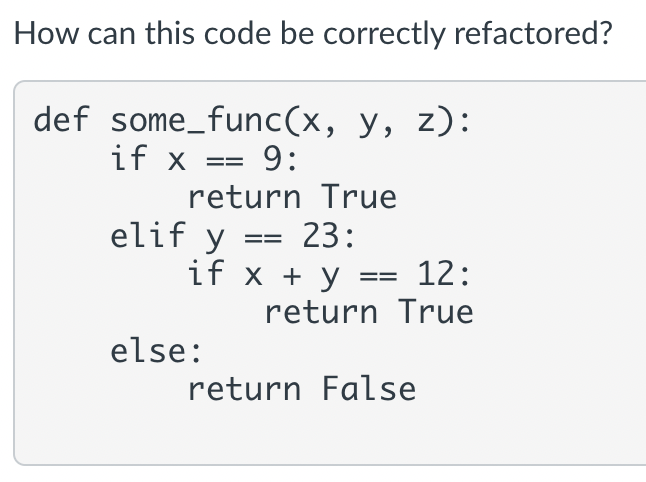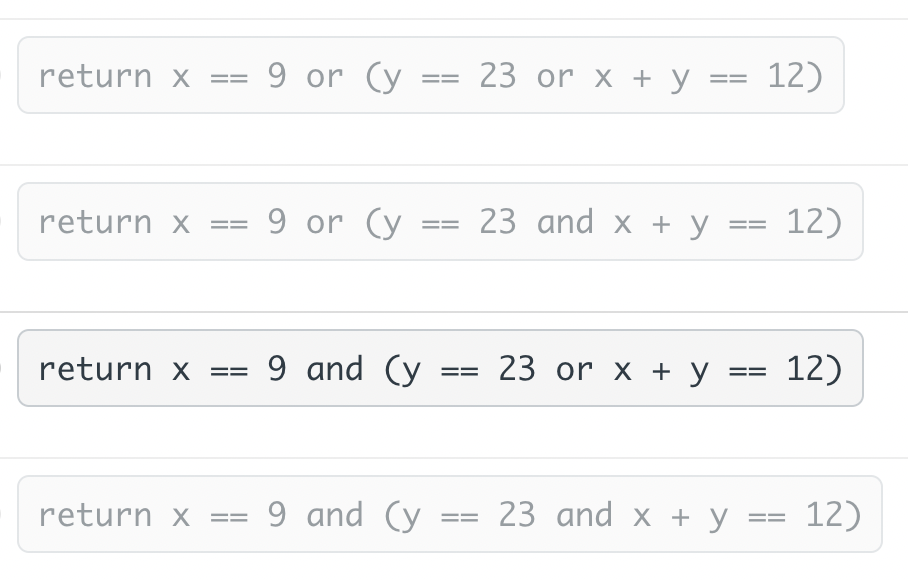Rating : ⭐⭐⭐⭐⭐
Price : \$10.99
Language:EN
Pages: 3
Words: 257

# Refactor and Simplify Code Assignment Answers Needed

How can this code be correctly refactored? def some_func (x,y,z) : if x==9 : return True elif y==23 : if x+y==12 : return True else: return False

choose options:# Step By Step Answers with Explanation

elif y == 23:

if x + y == 12:

def some_func(x, y, z):

return x == 9 and (y == 23 or x + y == 12)

- `x + y == 12`: It checks if the sum of `x` and `y` is equal to 12. If this condition is true, the overall expression is true.

The refactored code combines these conditions using `and` and `or` operators to achieve the same logic as the original code but in a more concise and readable manner.

How It Works